# 3.5 Lewis symbols and structures  (Page 2/15)

 Page 2 / 15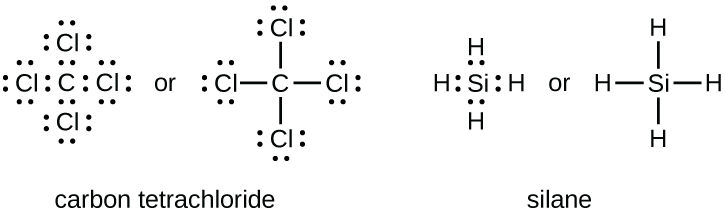Group 15 elements such as nitrogen have five valence electrons in the atomic Lewis symbol: one lone pair and three unpaired electrons. To obtain an octet, these atoms form three covalent bonds, as in NH 3 (ammonia). Oxygen and other atoms in group 16 obtain an octet by forming two covalent bonds: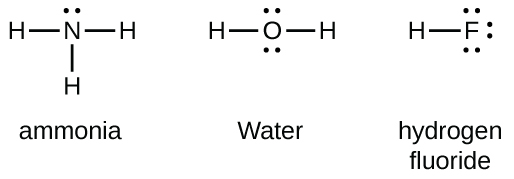## Double and triple bonds

As previously mentioned, when a pair of atoms shares one pair of electrons, we call this a single bond. However, a pair of atoms may need to share more than one pair of electrons in order to achieve the requisite octet. A double bond    forms when two pairs of electrons are shared between a pair of atoms, as between the carbon and oxygen atoms in CH 2 O (formaldehyde) and between the two carbon atoms in C 2 H 4 (ethylene):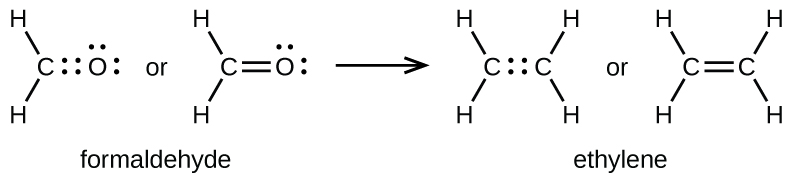A triple bond    forms when three electron pairs are shared by a pair of atoms, as in carbon monoxide (CO) and the cyanide ion (CN ):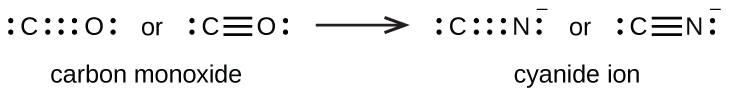## Writing lewis structures with the octet rule

For very simple molecules and molecular ions, we can write the Lewis structures by merely pairing up the unpaired electrons on the constituent atoms. See these examples: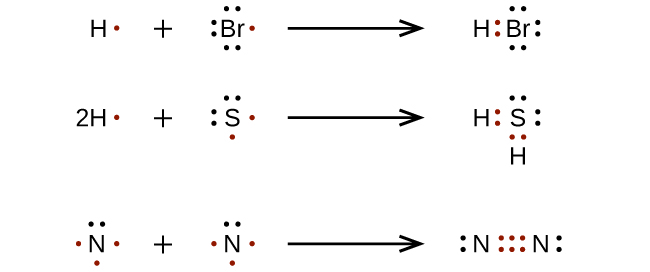For more complicated molecules and molecular ions, it is helpful to follow the step-by-step procedure outlined here:

1. Determine the total number of valence (outer shell) electrons. For cations, subtract one electron for each positive charge. For anions, add one electron for each negative charge.
2. Draw a skeleton structure of the molecule or ion, arranging the atoms around a central atom. (Generally, the least electronegative element should be placed in the center.) Connect each atom to the central atom with a single bond (one electron pair).
3. Distribute the remaining electrons as lone pairs on the terminal atoms (except hydrogen), completing an octet around each atom.
4. Place all remaining electrons on the central atom.
5. Rearrange the electrons of the outer atoms to make multiple bonds with the central atom in order to obtain octets wherever possible.

Let us determine the Lewis structures of SiH 4 , ${\text{CHO}}_{2}{}^{\text{−}},$ NO + , and OF 2 as examples in following this procedure:

1. Determine the total number of valence (outer shell) electrons in the molecule or ion.
• For a molecule, we add the number of valence electrons on each atom in the molecule:
$\begin{array}{l}\\ \phantom{\rule{0.8em}{0ex}}{\text{SiH}}_{4}\\ \phantom{\rule{0.8em}{0ex}}\text{Si: 4 valence electrons/atom}\phantom{\rule{0.2em}{0ex}}×\phantom{\rule{0.2em}{0ex}}\text{1 atom}=4\\ \underset{¯}{+\phantom{\rule{0.2em}{0ex}}\text{H: 1 valence electron/atom}\phantom{\rule{0.2em}{0ex}}×\phantom{\rule{0.2em}{0ex}}\text{4 atoms}=4}\\ \\ \phantom{\rule{16.0em}{0ex}}=\text{8 valence electrons}\end{array}$
• For a negative ion , such as ${\text{CHO}}_{2}{}^{\text{−}},$ we add the number of valence electrons on the atoms to the number of negative charges on the ion (one electron is gained for each single negative charge):
$\begin{array}{l}\\ \phantom{\rule{0.5em}{0ex}}{\text{CHO}}_{2}{}^{\text{−}}\\ \phantom{\rule{0.48em}{0ex}}\text{C: 4 valence electrons/atom}\phantom{\rule{0.2em}{0ex}}×\phantom{\rule{0.2em}{0ex}}\text{1 atom}=4\\ \phantom{\rule{0.8em}{0ex}}\text{H: 1 valence electron/atom}\phantom{\rule{0.2em}{0ex}}×\phantom{\rule{0.2em}{0ex}}\text{1 atom}=1\\ \phantom{\rule{0.05em}{0ex}}\text{O: 6 valence electrons/atom}\phantom{\rule{0.2em}{0ex}}×\phantom{\rule{0.2em}{0ex}}\text{2 atoms}=12\\ \underset{¯}{+\phantom{\rule{6.5em}{0ex}}\text{1 additional electron}=1}\\ \\ \phantom{\rule{15.50em}{0ex}}=\text{18 valence electrons}\end{array}$
• For a positive ion , such as NO + , we add the number of valence electrons on the atoms in the ion and then subtract the number of positive charges on the ion (one electron is lost for each single positive charge) from the total number of valence electrons:
$\begin{array}{}\\ \\ \phantom{\rule{0.1em}{0ex}}{\text{NO}}^{+}\\ \text{N: 5 valence electrons/atom}\phantom{\rule{0.2em}{0ex}}×\phantom{\rule{0.2em}{0ex}}\text{1 atom}=5\\ \\ \phantom{\rule{0.4em}{0ex}}\text{O: 6 valence electron/atom}\phantom{\rule{0.2em}{0ex}}×\phantom{\rule{0.2em}{0ex}}\text{1 atom}=6\\ \phantom{\rule{0.35em}{0ex}}\underset{¯}{+\phantom{\rule{0.5em}{0ex}}\text{−1 electron (positive charge)}\phantom{\rule{1.8em}{0ex}}=-1}\\ \\ \phantom{\rule{15.02em}{0ex}}=\text{10 valence electrons}\end{array}$
• Since OF 2 is a neutral molecule, we simply add the number of valence electrons:
$\begin{array}{l}\\ \phantom{\rule{0.8em}{0ex}}{\text{OF}}_{\text{2}}\\ \phantom{\rule{1.25em}{0ex}}\text{O: 6 valence electrons/atom}\phantom{\rule{0.2em}{0ex}}×\phantom{\rule{0.2em}{0ex}}\text{1 atom}=6\\ \phantom{\rule{0.1em}{0ex}}\underset{¯}{+\phantom{\rule{0.2em}{0ex}}\text{F: 7 valence electrons/atom}\phantom{\rule{0.2em}{0ex}}×\phantom{\rule{0.2em}{0ex}}\text{2 atoms}=14}\\ \phantom{\rule{16.28em}{0ex}}=\text{20 valence electrons}\end{array}$
2. Draw a skeleton structure of the molecule or ion, arranging the atoms around a central atom and connecting each atom to the central atom with a single (one electron pair) bond. (Note that we denote ions with brackets around the structure, indicating the charge outside the brackets:)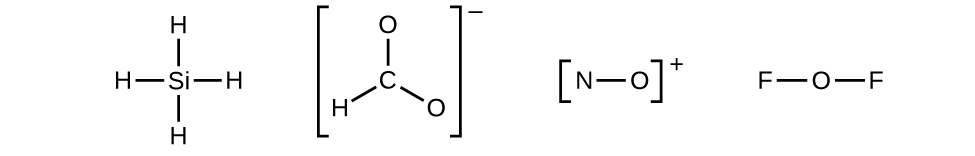When several arrangements of atoms are possible, as for ${\text{CHO}}_{2}{}^{\text{−}},$ we must use experimental evidence to choose the correct one. In general, the less electronegative elements are more likely to be central atoms. In ${\text{CHO}}_{2}{}^{\text{−}},$ the less electronegative carbon atom occupies the central position with the oxygen and hydrogen atoms surrounding it. Other examples include P in POCl 3 , S in SO 2 , and Cl in ${\text{ClO}}_{4}{}^{\text{−}}.$ An exception is that hydrogen is almost never a central atom. As the most electronegative element, fluorine also cannot be a central atom.
3. Distribute the remaining electrons as lone pairs on the terminal atoms (except hydrogen) to complete their valence shells with an octet of electrons.
• There are no remaining electrons on SiH 4 , so it is unchanged: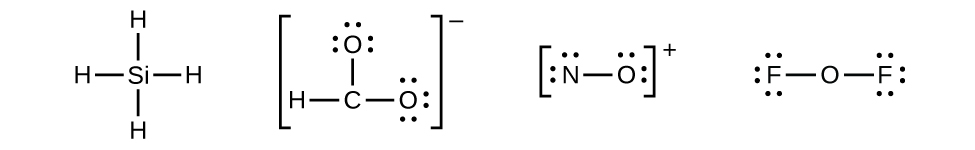4. Place all remaining electrons on the central atom.
• For SiH 4 , ${\text{CHO}}_{2}{}^{\text{−}},$ and NO + , there are no remaining electrons; we already placed all of the electrons determined in Step 1.
• For OF 2 , we had 16 electrons remaining in Step 3, and we placed 12, leaving 4 to be placed on the central atom: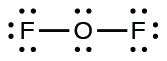5. Rearrange the electrons of the outer atoms to make multiple bonds with the central atom in order to obtain octets wherever possible.
• SiH 4 : Si already has an octet, so nothing needs to be done.
• ${\text{CHO}}_{2}{}^{\text{−}}:$ We have distributed the valence electrons as lone pairs on the oxygen atoms, but the carbon atom lacks an octet: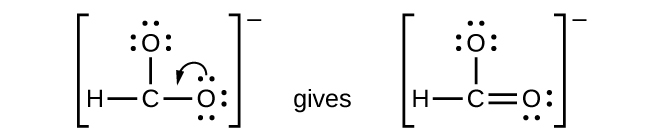• NO + : For this ion, we added eight valence electrons, but neither atom has an octet. We cannot add any more electrons since we have already used the total that we found in Step 1, so we must move electrons to form a multiple bond:This still does not produce an octet, so we must move another pair, forming a triple bond:• In OF 2 , each atom has an octet as drawn, so nothing changes.

how can chip be made from sand
are nano particles real
yeah
Joseph
Hello, if I study Physics teacher in bachelor, can I study Nanotechnology in master?
no can't
Lohitha
where we get a research paper on Nano chemistry....?
nanopartical of organic/inorganic / physical chemistry , pdf / thesis / review
Ali
what are the products of Nano chemistry?
There are lots of products of nano chemistry... Like nano coatings.....carbon fiber.. And lots of others..
learn
Even nanotechnology is pretty much all about chemistry... Its the chemistry on quantum or atomic level
learn
da
no nanotechnology is also a part of physics and maths it requires angle formulas and some pressure regarding concepts
Bhagvanji
hey
Giriraj
Preparation and Applications of Nanomaterial for Drug Delivery
revolt
da
Application of nanotechnology in medicine
has a lot of application modern world
Kamaluddeen
yes
narayan
what is variations in raman spectra for nanomaterials
ya I also want to know the raman spectra
Bhagvanji
I only see partial conversation and what's the question here!
what about nanotechnology for water purification
please someone correct me if I'm wrong but I think one can use nanoparticles, specially silver nanoparticles for water treatment.
Damian
yes that's correct
Professor
I think
Professor
Nasa has use it in the 60's, copper as water purification in the moon travel.
Alexandre
nanocopper obvius
Alexandre
what is the stm
is there industrial application of fullrenes. What is the method to prepare fullrene on large scale.?
Rafiq
industrial application...? mmm I think on the medical side as drug carrier, but you should go deeper on your research, I may be wrong
Damian
How we are making nano material?
what is a peer
What is meant by 'nano scale'?
What is STMs full form?
LITNING
scanning tunneling microscope
Sahil
how nano science is used for hydrophobicity
Santosh
Do u think that Graphene and Fullrene fiber can be used to make Air Plane body structure the lightest and strongest. Rafiq
Rafiq
what is differents between GO and RGO?
Mahi
what is simplest way to understand the applications of nano robots used to detect the cancer affected cell of human body.? How this robot is carried to required site of body cell.? what will be the carrier material and how can be detected that correct delivery of drug is done Rafiq
Rafiq
if virus is killing to make ARTIFICIAL DNA OF GRAPHENE FOR KILLED THE VIRUS .THIS IS OUR ASSUMPTION
Anam
analytical skills graphene is prepared to kill any type viruses .
Anam
Any one who tell me about Preparation and application of Nanomaterial for drug Delivery
Hafiz
what is Nano technology ?
write examples of Nano molecule?
Bob
The nanotechnology is as new science, to scale nanometric
brayan
nanotechnology is the study, desing, synthesis, manipulation and application of materials and functional systems through control of matter at nanoscale
Damian
how did you get the value of 2000N.What calculations are needed to arrive at it
Privacy Information Security Software Version 1.1a
Good
Berger describes sociologists as concerned with
what is hormones?
Wellington
how do you find theWhat are the wavelengths and energies per photon of two lines
The eyes of some reptiles are sensitive to 850 nm light. If the minimum energy to trigger the receptor at this wavelength is 3.15 x 10-14 J, what is the minimum number of 850 nm photons that must hit the receptor in order for it to be triggered?
A teaspoon of the carbohydrate sucrose contains 16 calories, what is the mass of one teaspoo of sucrose if the average number of calories for carbohydrate is 4.1 calories/g?
4. On the basis of dipole moments and/or hydrogen bonding, explain in a qualitative way the differences in the boiling points of acetone (56.2 °C) and 1-propanol (97.4 °C), which have similar molar masses
Calculate the bond order for an ion with this configuration: (?2s)2(??2s)2(?2px)2(?2py,?2pz)4(??2py,??2pz)3
Which of the following will increase the percent of HF that is converted to the fluoride ion in water? (a) addition of NaOH (b) addition of HCl (c) addition of NaF

#### Get Jobilize Job Search Mobile App in your pocket Now!By Caitlyn GobbleByBy OpenStaxBy Nicole DuquetteBy Ellie BanfieldBy OpenStaxBy Saylor FoundationBy OpenStaxBy OpenStaxBy OpenStax